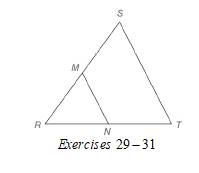Chapter 4.2, Problem 31EElementary Geometry For College St...

7th Edition
Alexander + 2 others
ISBN: 9781337614085

Solutions

Chapter
SectionElementary Geometry For College St...

7th Edition
Alexander + 2 others
ISBN: 9781337614085
Textbook Problem

In Exercises 29 to 31, M and N are the midpoints of sides R S ¯ and R T ¯ of ∆ R S T , respectively. Given: R M = R N = 2 x + 1   S T = 5 x - 3   m ∠ R = 60 ° Find: x , R M , and S TTo determine

To find:

x, RM, and ST

For RM=RN=2x+1

ST=5x-3

mR=60°

Explanation

Calculation:

Given,

RM=RN=2x+1

ST=5x-3

mR=60°

M and N are the midpoints of sides RS¯ and RT¯ OF RST respectively.

A line segment that joins the mid points of two sides of a triangle is parallel to the third sides and has a length equal to one-half the length of the third side.

Thus,

MN=12ST

MN=125x-3

Now, in RMN we have

mR=60°

But, RM=RN

Since, angle opposite to the equal sides are equal in measure,

mM=mN=xsay

By the angle sum property of a triangle in RMN

R+M+N=18O°

60°+x+x=180°

60°+2x=180°

Add; -60° on both sides,

60°+2x+-60°=180°+-60°

2x+60°+-60°=180°-60°

2x=120°

Divide; by 2 on both sides,

2x2=120°2

x=60°

Thus,

R=M=N=60°

Hence, RMN is an equilateral triangle

Still sussing out bartleby?

Check out a sample textbook solution.

See a sample solution

The Solution to Your Study Problems

Bartleby provides explanations to thousands of textbook problems written by our experts, many with advanced degrees!

Get Started

Evaluate the integrals in Problems 7-36. Check your results by differentiation. 23.

Mathematical Applications for the Management, Life, and Social Sciences

Expand each expression in Exercises 122. (2x3)(2x+3)

Finite Mathematics and Applied Calculus (MindTap Course List)

Find for x = 3t2 + 1, y = t6 + 6t5. t4 + 5t3 4t3 + 15t2

Study Guide for Stewart's Multivariable Calculus, 8th